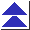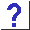# 3. Expressions

3.1 Why doesn't this code:

`a[i] = i++;`
work?

3.2 Under my compiler, the code

```int i = 7;
printf("%d\n", i++ * i++);```
prints 49. Regardless of the order of evaluation, shouldn't it print 56?

3.3 I've experimented with the code

```int i = 3;
i = i++;```
on several compilers. Some gave i the value 3, and some gave 4. Which compiler is correct?

3.3b Here's a slick expression:

`a ^= b ^= a ^= b`
It swaps a and b without using a temporary.

3.4 Can I use explicit parentheses to force the order of evaluation I want, and control these side effects? Even if I don't, doesn't precedence dictate it?

3.5 But what about the && and || operators?
I see code like ``while((c = getchar()) != EOF && c != '\n')'' ...

3.6 Is it safe to assume that the right-hand side of the && and || operators won't be evaluated if the left-hand side determines the outcome?

3.7 Why did

`printf("%d %d", f1(), f2());`
call f2 first? I thought the comma operator guaranteed left-to-right evaluation.

3.8 How can I understand complex expressions like the ones in this section, and avoid writing undefined ones? What's a ``sequence point''?

3.9 So if I write

`a[i] = i++;`
and I don't care which cell of a[] gets written to, the code is fine, and i gets incremented by one, right?

3.10a People keep saying that the behavior of i = i++ is undefined, but I just tried it on an ANSI-conforming compiler, and got the results I expected.

3.10b People told me that if I evaluated an undefined expression, or accessed an uninitialized variable, I'd get a random, garbage value. But I tried it, and got zero. What's up with that?

3.11 How can I avoid these undefined evaluation order difficulties if I don't feel like learning the complicated rules?

3.12a What's the difference between ++i and i++?

3.12b If I'm not using the value of the expression, should I use ++i or i++ to increment a variable?

3.13 I need to check whether one number lies between two others. Why doesn't

`if(a < b < c)`
work?

3.14 Why doesn't the code

```int a = 1000, b = 1000;
long int c = a * b;```
work?

3.14b How can I ensure that integer arithmetic doesn't overflow?

3.15 Why does the code

```double degC, degF;
degC = 5 / 9 * (degF - 32);```
keep giving me 0?

3.16 I have a complicated expression which I have to assign to one of two variables, depending on a condition. Can I use code like this?

```	((condition) ? a : b) = complicated_expression;
```

3.17 I have some code containing expressions like

`a ? b = c : d`
and some compilers are accepting it but some are not.

3.18 What does the warning ``semantics of `>' change in ANSI C'' mean?

3.19 What's the difference between the ``unsigned preserving'' and ``value preserving'' rules?